Cinderpelt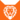狮族 (Q646)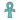转世为炭心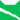雷族 学徒雷族 巫医雷族 首席巫医
（继任自黄牙 (Q2724)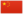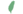炭崽非官 Cinderkit  小煤非官
 炭爪 Cinderpaw  煤掌
 炭毛 Cinderpelt  煤皮

Q3157：雷族母猫，自《呼唤野性》登场的角色

## 细节

### 外貌

• 她是一只身形娇小、:117淡蓝色眼睛:238的烟:45灰色母猫，:猫物表皮毛光滑。:30
• 她的灰色皮毛曾被描述为旧火中的余烬。:263

### 趣闻

• 她和兄弟蕨毛成为学徒时都未满六个月。:93
• 侄女炭心的名字是为了纪念她。:27
• 侄女炭心的外貌和她极为相似。:21章
• 侄女炭心是她的转世。:217
• 然而在《群星之战》中，她在炭心并未死去的情况下脱离了她的身体。比起转世reincarnation），这看上去更像是她附身possession）在炭心身上，因为两者并非同一个灵魂。

## 登场书目

• 呼唤野性 (Q57)[配角][首次登场🐱]
• 寒冰烈火 (Q59)[配角]
• 疑云重重 (Q60)[配角]
• 风起云涌 (Q65)[配角]
• 险路惊魂 (Q66)[配角]
• 力挽狂澜 (Q67)[配角]
• 午夜追踪 (Q139)[配角]
• 新月危机 (Q140)[配角]
• 重现家园 (Q141)[配角]
• 星光指路 (Q142)[配角]
• 黄昏战争 (Q143)[配角][死亡时刻✝]
• 日落和平 (Q144)[对话提及]
• 预视力量 (Q152)[对话提及]
• 暗河汹涌 (Q153)[对话提及]
• 驱逐之战 (Q154)[对话提及]
• 天蚀遮月 (Q155)[对话提及]
• 暗夜长影 (Q156)[对话提及]
• 拂晓之光 (Q157)[对话提及]
• 第四学徒 (Q158)[对话提及]
• 暗夜密语 (Q160)[对话提及]
• 武士归来 (Q162)[仅于幻象]
• 群星之战 (Q163)[配角]
• 烈焰焚河 (Q174)[配角]
• 叠影重障 (Q3624)[对话提及]
• 火星的探索 (Q176)[配角]
• 黄牙的秘密 (Q180)[配角]
• 黑莓星的风暴 (Q182)[对话提及]
• 蛾飞的幻象 (Q183)[仅于幻象]
• 松鼠飞的希冀 (Q3561)[配角]
• 灰条的誓言 (Q4057)[对话提及]
• 一星的告解 (Q4826)[对话提及]
• 虎掌的愤怒 (Q190)[配角]
• 叶池的祈愿 (Q191)[对话提及]
• 红尾的恩债 (Q3565)[配角]
• 蛾翅的秘密 (Q3628)[配角]
• 黑脚的审判 (Q4336)[仅于回忆]
• 逐出影族 (Q4769)[配角]
• 武士回归 (Q209)[仅于回忆]
• 族群救星 (Q214)[配角]
• 族群的秘密 (Q199)[追溯修订]
• 族群的猫 (Q200)[配角]
• 族群的战争 (Q202)[对话提及]
• 终极指南 (Q204)[配角]
• ## 周边

更多资料：炭毛/周边

【系列2】炭毛和亮心
• 系列2——炭毛和亮心黑星和带松鸦羽乌爪沙风
• 这对雷族姐妹花历经了艰巨的挑战并取得了胜利。炭毛成为武士的梦想在她弄断腿的那一天破灭了，但她在她巫医的新角色中有着杰出的表现。同时，亮心证明自己是一名伟大武士的决心几乎令她付出了生命的代价——虽然她也将克服她的伤痛，并在族群的荣耀中赢得一席之地。
• 长约7厘米。
• 一组约12.99美金；整套三组约38.97美金。

## 脚注

1. 《呼唤野性》中提到被绑架的幼崽之一为灰色母猫，炭毛是唯一符合此描述的猫；此外，她和她的三个同窝手足还出现在了《逐出影族》中爪脸绑架雷族幼崽的片段中。然而她又曾以旁观者的口吻描述绑架事件，无法确认这是剧情衔接失误还是她当时太过年幼而出现了记忆偏差。
2. 根据官方发售的迷你公仔，她的右后脚扭曲变形；但同为官方发售的绒毛娃娃，变形的却是她的左后脚。

## 参考文献

这篇文章基于CC BY-SA 3.0许可使用了猫武士维基（英语）Cinderpelt一文中的部分内容。